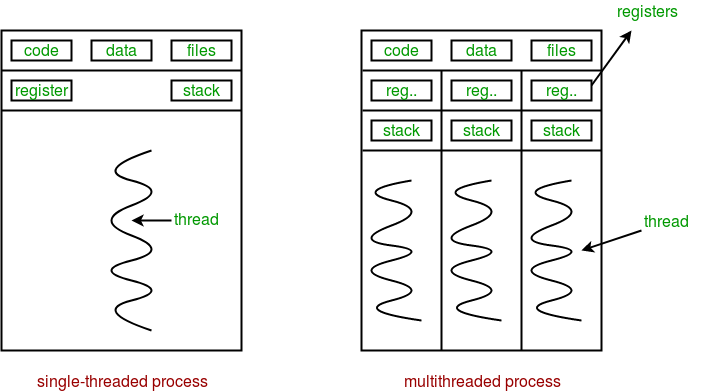Total Post:113

Points:791

483  View(s)
Ratings:
Rate this:

What is the concept of Multi threading. It would be better for me if anyone given answer in Python with example .

1.Multi threading means multi processing and multitasking.You can speed your code up through multi-thread because python having multi threading packages. This happens very quickly so to the human eye it may seem like your threads are executing in parallel, but they are really just taking turns using the same CPU core.

def print_cube(n):
print("Cube: {}".format(n*n*n))
def print_square(n):
print("Square: {}".format(n*n))
if __name__ == "__main__":
t1.start() // starting the thread 1
t2.start()
t1.join() // exicute the thread 1
t2.join()
print("Done!")

You can understand this by this image :Happy Coding :)